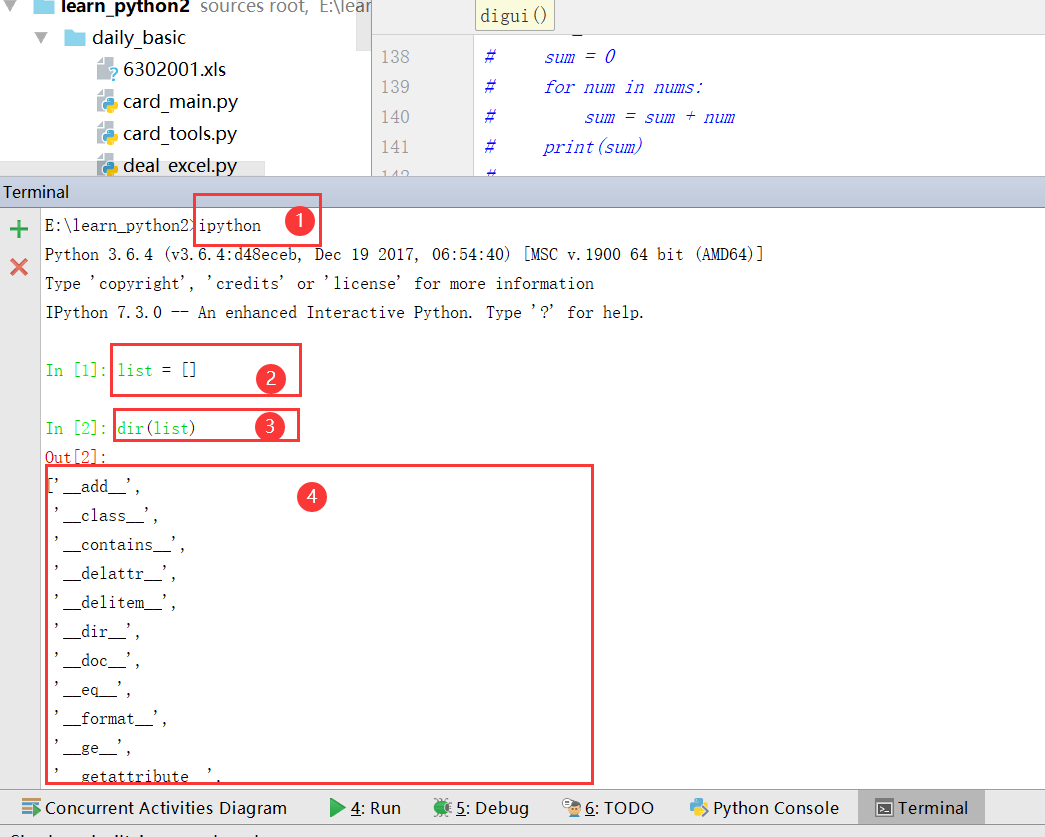# 1. dir 命令查看内置函数和方法

• 打开 pycharm 中的 ipython 编译器
• 创建一个对象 （文中创建了一个列表对象 ``` list ```
• 使用 ``` dir ``` 命令来查看对象的所有内置函数和方法# 2. 不同方法的作用

## 2.1 ``` __init__() ``` 方法：

• 在定义一个类 class 的时候，如果想为这个 class 添加一些属性，需要先使用 __init__() 方法来进行初始化，例如：
``````class Cat:

def __init__(self):
self.name = "Tom"

tom = Cat()
print(tom.name)

----> 输出结果： Tom
``````

## 2.2 ``` __del__() ``` 方法：

``````class Cat:

def __init__(self):
self.name = "Tom"
print("hello")

def __del__(self):
print("再见")

tom = Cat()

------> 输出结果：hello
再见
``````

## 2.3 ``` __str__() ``` 方法：

``````class Cat:

def __init__(self):
self.name = "Tom"

tom = Cat()
print(tom)

-----> 输出结果： <__main__.Cat object at 0x0000020139FD67B8>
``````

``````class Cat:

def __init__(self):
self.name = "Tom"

def __str__(self):
return "我是一只猫"

tom = Cat()
print(tom)

-----> 输出结果：我是一只猫

``````